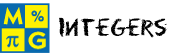IntegersThis page lists the Learning Objectives for all lessons in Unit 5.

Introduction to Integers

The student will be able to:

• Define the set of integers, positive numbers, negative numbers, opposites and signs.
• List real-life situations involving opposites.
• Identify an integer to represent a given real-life situation.
• Identify the opposite of an integer.
• Indicate an integer from a number line.
• Differentiate between a positive integer and a negative integer.
• List real-life situations involving integer opposites.
• Recognize that a positive integer can be written with or without a sign.
• Apply integer concepts to complete five interactive exercises.

Absolute Value

The student will be able to:

• Define absolute value.
• Describe the relationship between distance and absolute value.
• Identify the absolute value of an integer using the number line.
• Identify the absolute value of each integer in a given set of numbers.
• Express the absolute value of an integer using the proper notation.
• Determine all integers that satisfy a given condition for absolute value.
• Apply absolute value concepts to complete five interactive exercises.

Comparing and Ordering Integers

The student will be able to:

• Differentiate between the inequality symbols < and >.
• Compare two integers, using the proper inequality symbol.
• Order a set of integers from least to greatest.
• Order a set of integers from greatest to least.
• Recognize that Integers get smaller in value as you move to the left, and larger as you move to the right on the number line.
• Apply procedures for comparing and ordering integers to complete five interactive exercises.

The student will be able to:

• Review the definition of absolute value.
• Describe the relationship between a negative integer and owing money.
• Recognize that the sum of two negative integers is a negative integer.
• Perform addition of two negative integers.
• Recognize that the sum of two positive integers is a positive integer.
• Perform addition of two positive integers.
• Describe the procedure for adding integers with unlike signs.
• Describe how absolute value concepts are used in this procedure.
• Perform addition of integers with unlike signs by applying the procedure.
• Differentiate between the sign of an integer and the operation being performed.
• Recognize that the sum of any integer and its opposite is equal to zero.
• Apply the procedures for adding integers to complete five interactive exercises.

Subtracting Integers

The student will be able to:

• Perform subtraction of integers using the number line.
• Recognize the need for an arithmetic procedure for subtracting large integers.
• Describe the arithmetic procedure for subtracting integers.
• Perform subtraction of integers using the arithmetic procedure.
• Describe how to convert an integer subtraction problem into an addition problem.
• Apply the procedure for subtracting integers to complete five interactive exercises.

Multiplying Integers

The student will be able to:

• Restate that the product of two integers with unlike signs is a negative integer.
• Restate that the product of two integers with like signs is a positive integer.
• Define the Associative Law of Multiplication.
• Perform multiplication of two integers with like signs.
• Perform multiplication of two integers with unlike signs.
• Recognize that the Associative Law of Multiplication applies to integer multiplication.
• Perform multiplication of three integers, two at a time, applying the rules for multiplying integers.
• Recognize that when multiplying three integers, one can multiply the product of any two by the third.
• Apply the procedures for integer multiplication to complete five interactive exercises.

Dividing Integers

The student will be able to:

• Restate that the quotient of two integers with unlike signs is a negative integer.
• Restate that the quotient of two integers with like signs is a positive integer.
• Perform division of two integers with like signs.
• Perform division of two integers with unlike signs.
• Describe the procedure for dividing integers with like signs.
• Describe the procedure for dividing integers with unlike signs.
• Apply the procedures for integer division to complete five interactive exercises.

Operations With Integers

The student will be able to:

• Review the rules and procedures for adding, subtracting, multiplying and dividing integers.
• Evaluate ten interactive exercises that provide mixed review of integer operations.
• Identify which procedure is needed for each exercise.
• Apply the procedures for integer operations to complete each exercise.
• Differentiate between the rules and procedures for all integer operations.

Practice Exercises

The student will be able to:

• Examine ten interactive exercises for all topics in this unit.
• Determine which concepts and procedures are needed to complete each practice exercise.
• Compute answers by applying appropriate formulas and procedures.
• Self-assess knowledge and skills acquired from this unit.

Challenge Exercises

The student will be able to:

• Evaluate ten interactive exercises with real-world word problems for all topics in this unit.
• Analyze each problem to identify the given information.
• Formulate a strategy for solving each problem.
• Apply strategies to solve routine and non-routine problems.
• Synthesize all information presented in this unit.
• Connect integers to the real world.
• Develop problem-solving skills.

Solutions

The student will be able to:

• Examine the solution for each exercise presented in this unit.
• Identify which solutions need to be reviewed.
• Compare solutions to completed exercises.
• Identify and evaluate incorrect answers.
• Amend and label original answers.
• Identify areas of strength and weakness.
• Decide which concepts, formulas and procedures need to be reviewed from this unit.

Order your CD today for Home or SchoolOR